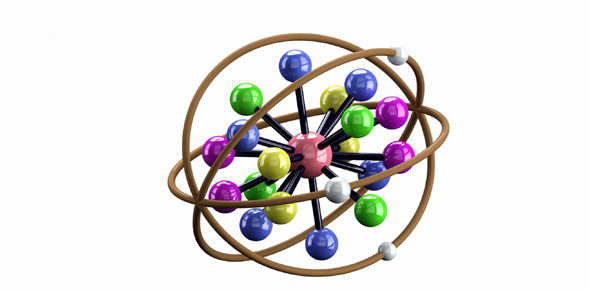Ionic Bonding Practice Quiz

7 Questions | Total Attempts: 4174SettingsA small, 7-question practice quiz on Ionic Bonding at www. Thechemwhiz. Piczo. Com

• 1.
When can ionic bonds be formed?
• A.

When there is a metal and a nonmetal.

• B.

When there are two solids.

• C.

When there are nonmetals.

• 2.
If in the process of ionic bonding, would Nitrogen be an cation or an anion?
• 3.
Lithium has a charge of +1 and Nitrogen has a charge of -3. What would be the name of the compound, in terms of letters AND in terms of chemical symbols? (Note: Subscripts cannot be used, so interpret the numbers in the chemical formulas as subscripts).
• A.

Lithium Nitride and LiN3

• B.

Trilithium Nitrogen and Li3N

• C.

Lithium Nitride and Li3N

• D.

Trilithium Nitride and LiN

• 4.
Nickel (II) has a charge of +2. Chlorine has a charge of -1. What is the name of the compound and what is the formula of the compound?
• A.

Nickel (II) Chloride and NiCl2

• B.

Nickel Chloride and NiCl2

• C.

Nickel Chlorine and NiCl

• D.

Nickle (II) Chloride and Ni2Cl

• 5.
Boron has a charge of +3 and Nitrogen has a charge of -3. What is the chemical compound name and formula?
• A.

Boron Nitrogen and BN

• B.

Uniboron Uninitrogen and BN

• C.

Boron Nitride and B3N3

• D.

Boron Nitride and BN

• 6.
Mercury (II) (Hg) has a charge of +2. Oxygen (O) has a charge of -2. What is the chemical compound name and formula?
• A.

Mercury (II) Oxide, HgO

• B.

Mercury Oxide, HgO

• C.

Mercury (II) Oxide, Hg2O

• D.

Mercury Oxygen, Hg2O

• 7.
Why are ionic compounds brittle?
• A.

Because they're solids.

• B.

Because when something hits it, a layer of ions is pushed so that they are next to ions of the same charge. They are repulsed by each other and break.

• C.

Because they're not strong bonds.

• D.

Because when something hits it, a layer of ions is pushed so that they are next to ions of the same charge. They are attracted to each other and break.

Related TopicsBack to top